# Core 1 Revision

Some basic points, examples and equations for some topics in Core 1.

Some of the symbols and text may not be shown, sorry!

?
• Created by: Rajvir
• Created on: 17-05-14 18:47

## Completing the Square - Sketching Graphs

Example 1

y = x^2 - 8x + 6   =>  y = (x-4)^2 -16 + 6   =>  y = (x-4)^2 -10

let f(x) = x^2

f(x-4) = (x-4)^2 <-- 4 units right parallel to x axis

f(x-4) - 10 = (x-4)^2 - 10 <--  10 units down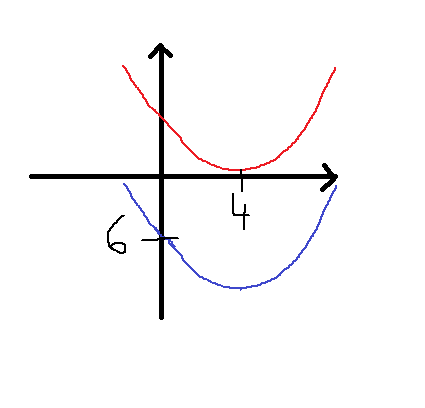sub x = 0 to see where it crosses x value
y = (0)^2 - 8(0) + 6 = 6

1 of 31

## Roots and the discriminant of a quadratic equation

ax^2 + bx + c = 0 ====> x = -b±√b^2 - 4ac
2a
b^2 - 4ac = discriminant

Example 1

x^2 - x - 6 =0 ===> a=1, b=-1, c=-6

-b±√b^2 - 4ac   ==> -(-1)±√(-1)^2 - 4(1)(-6)    ==>  1±25   ==>  1+5 and 1-5 ==> 3 and -2
2a                                2(1)                            2                2           2

==> 2 solutions => 2 different roots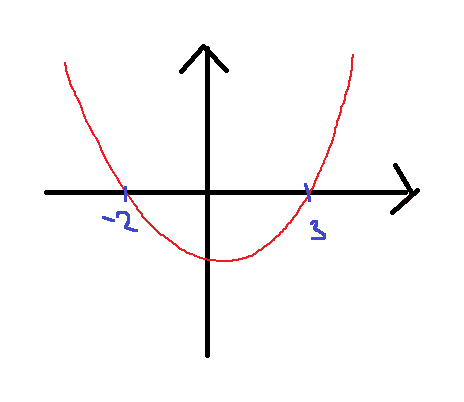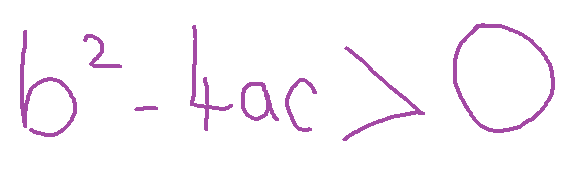2 of 31

## Roots and the discriminant of a quadratic equation

Example 2

x^2 - 10x + 25 = 0 ==> a=1, b=-10, c=25

-b±√b^2 - 4ac ==> -(-10)±√(-10)^2-4(1)(25) ==> 10±√0 ==> 10+0 and 10-0 ==> 5 and 5
2a                               2(1)                           2              2            2

1 solution = 1 root = equal roots ==> touches the curve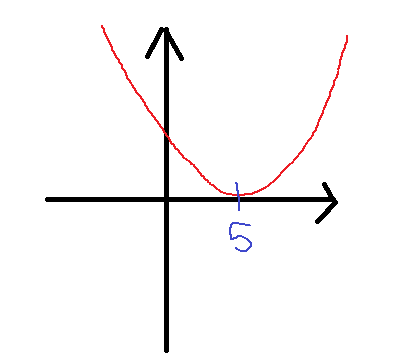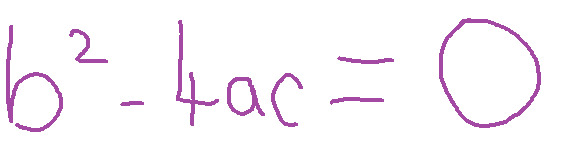3 of 31

## Roots and the discriminant of a quadratic equation

Example 3

2x^2 + 2x + 1 = 0 ==> a=2, b=2, c=1

-b±√b^2 - 4ac => -2±(2)^2-4(2)(1) => -2±-4  => CAN'T SQUARE -VE => no roots/solution
2a                        2(2)                   4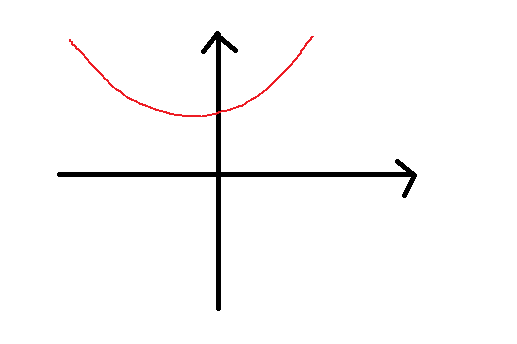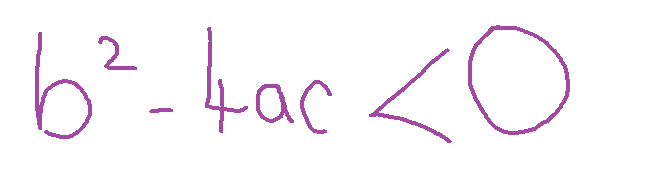4 of 31

## Roots and the discriminant of a quadratic equation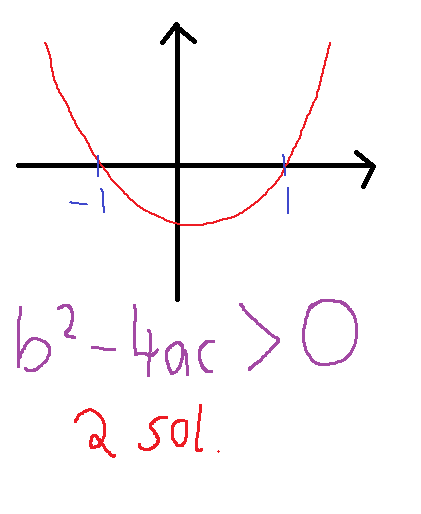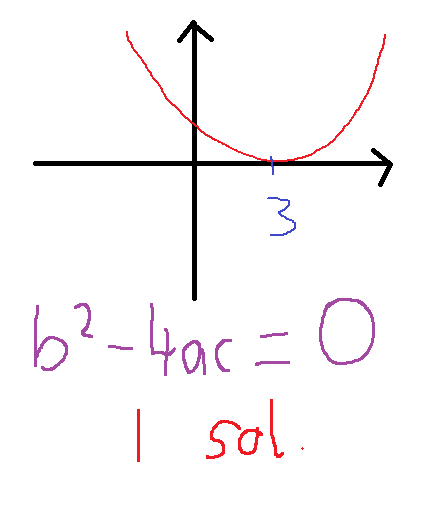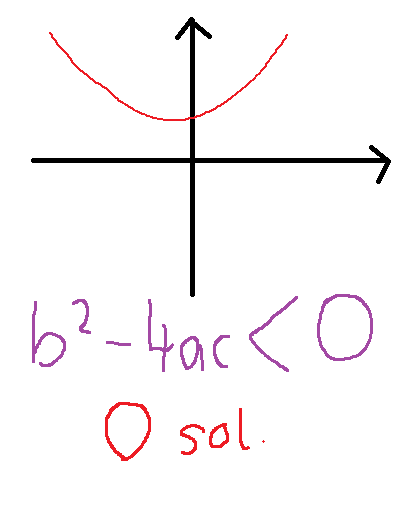5 of 31

## Polynomials

3x^2 + 5x +2 ==> quadratic polynomial - degree 2

5x^3 - 2x^2 + 1 ==> cubic polynomial - degree 3 (don't need every term e.g. x^1 missing here)

7x^4 - 3x^3 + 4x^2 - 2x ==> quartic polynomial - degree 4

Example 1

If p = 2x^3 - 5x +1 , q = x^4 - x , write in descending powers of x: i) p-q ii) pq

i) p-q = 2x^3 - 5x + 1 - (x^4 - x) = 2x^3 - 5x + 1 - x^4 + x = - x^4 + 2x^3 - 4x + 1

ii) pq = (2x^3 - 5x +1)(x^4 - x) = 2x^7  - 2x^4 - 5x^5 + 5x^2 + x^4 - x = 2x^7 - 5x^5 - x^4 + 5x^2 - x

6 of 31

## Graphs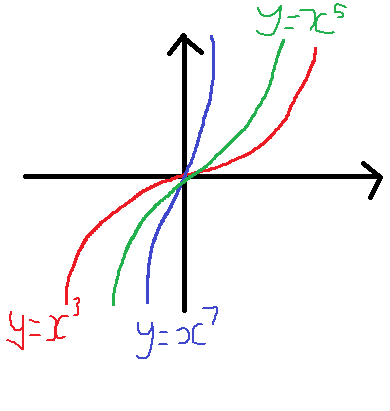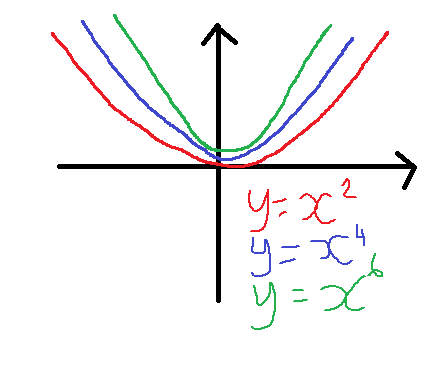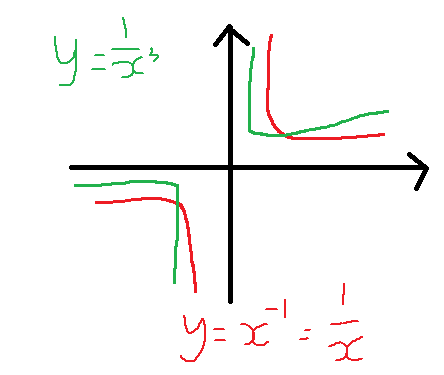7 of 31

## Translations

y = f(x-a) translates y = f(x) a units to the right

y = f(x+a) translates y = f(x) a units to the left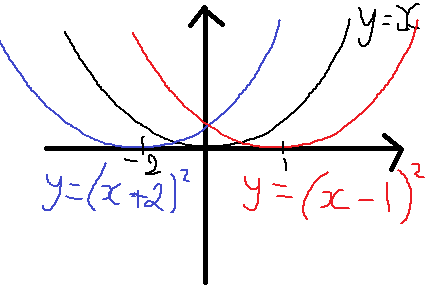y = (x+2)^2 = f(x+2) = 2 units left
y = (x-1)^2 = f(x-1) = 1 unit right

8 of 31

## Translations

y = f(x) - a translates y = f(x) a units down

y = f(x) + a translates y = f(x) a units up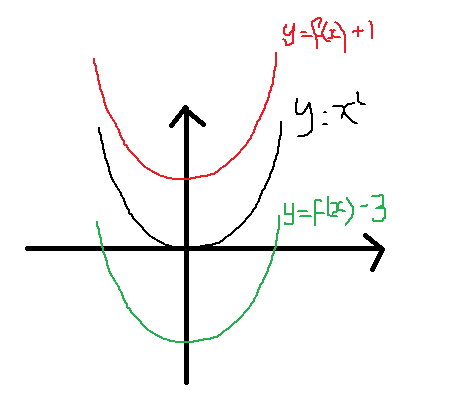y = f(x) = x^2
y = f(x) -3 = 3 down
y = f(x) +1 = 1 up

9 of 31

## Reflections

y = -f(x) reflects y= f(x) in the x axis

y = f(-x) reflects y = f(x) in the y axis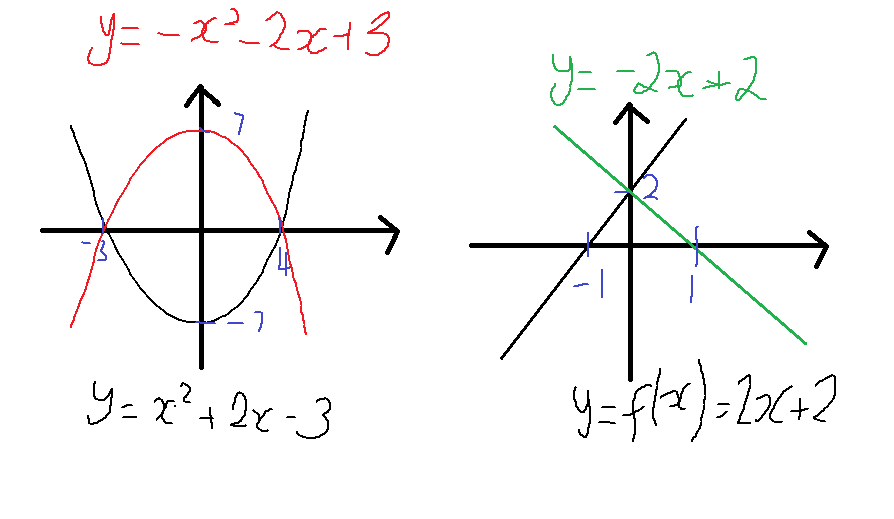10 of 31

## Stretches

y = af(x) stretches y = f(x) by scale factor a  parallel to the y axis

y = f(ax) stretches y = f(x) by a scale factor 1/a parallel to the x axis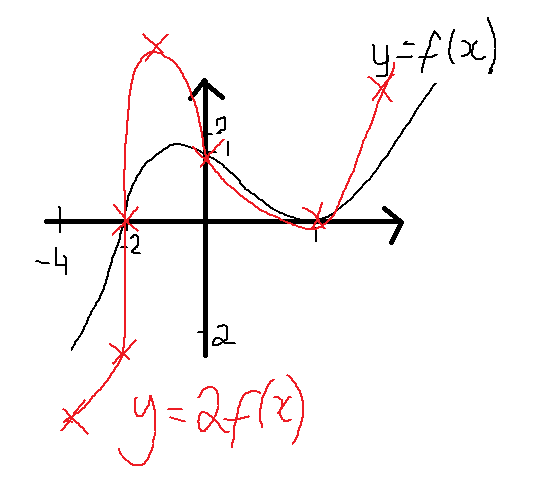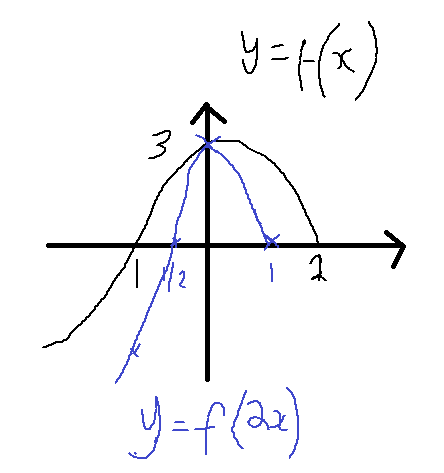some invarient points*

11 of 31

## Transformations of Graphs Rules

TRANSLATIONS

y = f(x-a) translates y = f(x) a units to the right

y = f(x+a) translates y = f(x) a units to the left

y = f(x) - a translates y = f(x) a units down

y = f(x) + a translates y = f(x) a units up

REFLECTIONS

y = -f(x) reflects y = f(x) in the x axis

y = f(-x) reflects y = f(x) in the y axis

STRETCHES

y = af(x) stretches y = f(x) by a scale factor of a parallel to the y axis

y = f(ax) stretches y = f(x) by a scale factor of 1/a parallel to the x axis

12 of 31

## Inequalities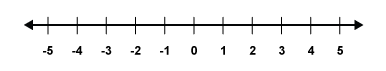if dividing by a negative number, reverse the inequality so it is true..

e.g. 2 < 6 ===> (divide by -2) ===> -1 > -3

if multiplying by a negative number, reverse the inequality for it to be true...

e.g 2 < 6 ===> (multiply by -2) ===> -4 > -12

13 of 31

## Inequalities - Double type

4 < 5x - 1 < x + 11

4 < 5x - 1 ////////// 5x - 1 < x + 11

5 < 5x /////////////// 5x < x + 12

1 < x ///////////////// 4x < 12

x > 1 ////////////////// x < 3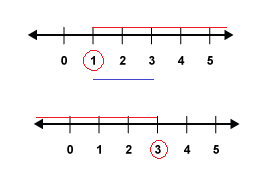1 < x < 3

14 of 31

## Sequences and Series

Recurrance Relationships

Example 1

6, 13, 27, 55

U1 = 6, U2 = 13, U3 = 27, U4 = 55 ==> Un+1 = 2Un + 1

Exam question

Write down the first five terms in the sequence Un+1 = 3Un^2 - 9 where U1 is 2.

when n = 1 => U2 = 2(U1)^2 - 9 = 3(2)^2 - 9 = 3
when n = 2 => U3 = 2(U2)^2 - 9 = 3(3)^2 - 9 = 18
when n = 3 => U4 = 2(U3)^2 - 9 = 3(18)^2 - 9 = 963
when n = 4 => U5 = 2(U4)^2 - 9 = 3(963)^2 - 9 = 2782098

sequence: 2, 3, 18, 963, 2782098

15 of 31

## Series Sigma Notation

Example 1

rth term in a sequence = 12/r
1st term => r = 1 => 12/1 = 12
2nd term => r = 2 => 12/2 = 6
3rd term => r = 3 => 12/3 = 4
4th term => r = 4 => 12/4 = 3

Series... 12 + 6 + 4 + 3 = 25 ==> sigma notation just means add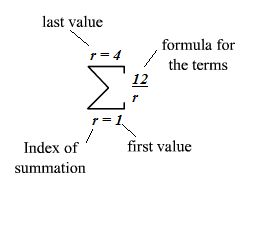16 of 31

## Series Sigma Notation 2

Example 1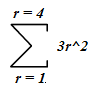3(1)^2 + 3(2)^2 + 3(3)^2 + 3(4)^2
= 3 [ (1)^2 + (2)^2 + (3)^2 + (4)^2] ===>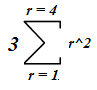= 3 [1+4+9+16] = 90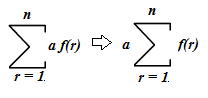17 of 31

## Arithmetic Sequences and Series - Progression

Example 1

6, 8, 10, 12 ==> common difference (d) = +2, first term (a) = 6
11, 8, 5, 3 ==> d = -3, a = 11

In general...

1st, 2nd,   3rd,   4th,       9th
a, a+d, a+2d, a+3d.... a+8d

nth term = a+(n-1)d

Example 2: For the sequence 5, 9, 13, 17... what is the 21st term?

a = 5, d = +4 n = 21

a+(n-1)d = 5+(21-1)x4 = 5+(20x4) = 5+80 = 85

18 of 31

## Arithmetic Sequences and Series - Progression

SUM

Sn = n/2[2a+(n-1)d] = n/2[a+l] where a = first term, d = common difference, l = last term

Example 1: 5, 9, 13, 17 => a = 5, d = 4, n = 21

Sn = n/2[2a+(n-1)d] = 21/2[2(5)+(21-1)x4] = 21/2[10+(20x4)] = 21/2 = 21x45 = 945

Sn = n/2[a+l] = 21/2[5+85] = 21/2 = 21x45 = 945

19 of 31

## Coordinate Geometry

* Gradient of a Line Segment
Gradient of line AB = 2 ======> Gradient of line CD = -5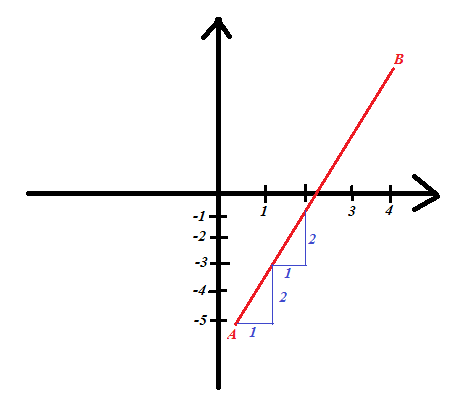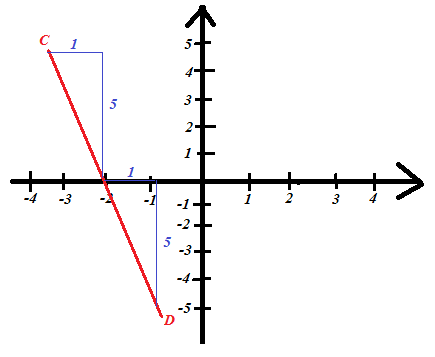CHANGE IN X

20 of 31

## Coordinate Geometry

* if two lines are parallel, their gradients are equal *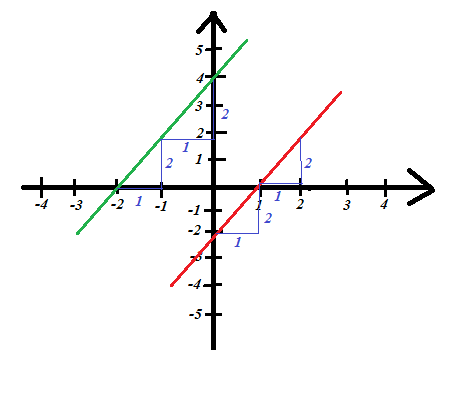gradient of the green line = 2
gradient of the red line = 2

21 of 31

## Coordinate Geometry

PERPENDICULAR LINES

* the gradient of a perpendicular line is the negative reciprocal of the first line *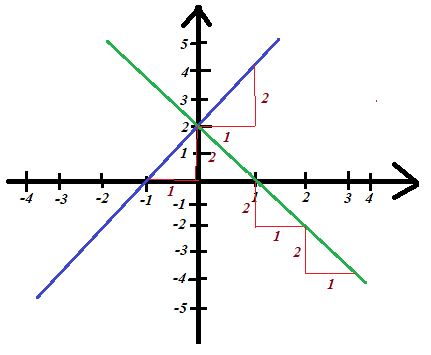grad of blue line = 2
grad of green line = -1/2

22 of 31

## Coordinate Geometry - y = mx + c

Example 1: y = 2x + 1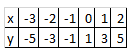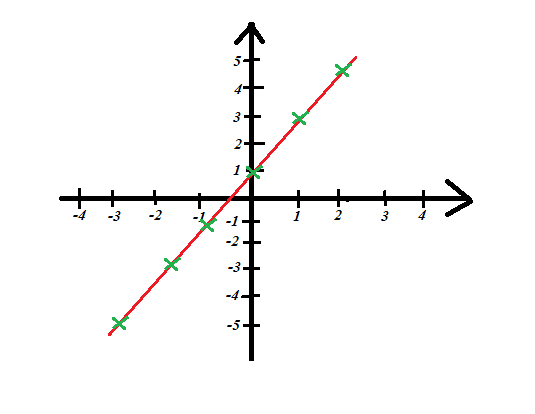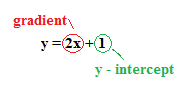23 of 31

## Coordinate Geometry - Distance between 2 points

Example 1

AB^2 = AC^2 +  BC^2
AB^2 = 8^2 + 6^2
AB^ = 64 + 36 = 100
AB = 100 = 10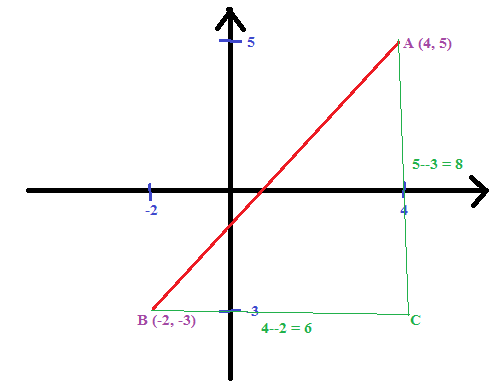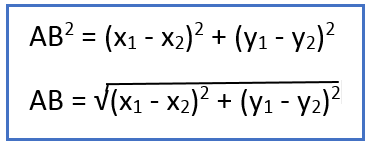24 of 31

## Coordinate Geometry - Midpoint of a line segment

Example 1

Midpoint of AB = (-2+3, 4+-2) = (1/2, 1)
2       2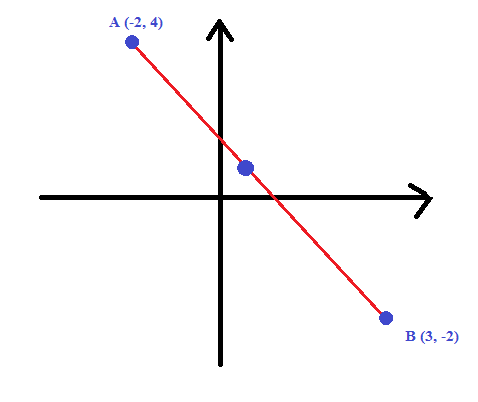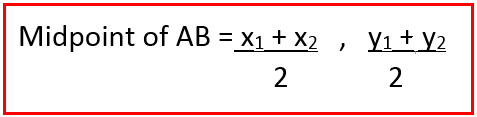25 of 31

## Coordinate Geometry - Equation of Parallel Line

Example 1: Find the equation of a line parallel to the line 3y - 2x - 6 = 0 and passing through the point (-1,-2)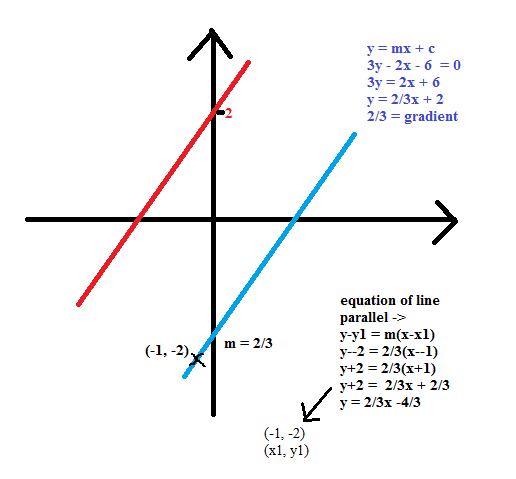26 of 31

## Coordinate Geometry - Equation of perp. bi sector

Example 1: Find the equation of the perpendicular bisector of the line joining the points A (3,5) and B (-2,-1)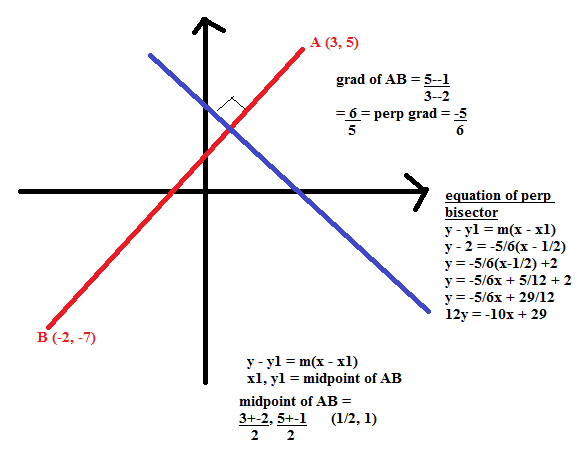27 of 31

## Coordinate Geometry - Intersections

Intersection of 2 straight lines:

SOLVE SIMULTANEOUSLY => get the point of intersection

Intersection of a line and U shaped curve (parabola):

MAKE THEM EQUAL EACHOTHER => get equation => solve = 2 values of x and 2 of y

Intersection of a tangent to curve (parabola):

MAKE THEM EQUAL EACHOTHER => b2-4ac = 0 => solve => 1 root

Intersection of a line and hyperbola (1/x curves):

MAKE THEM EQUAL EACHOTHER => solve equation

28 of 31

## Nature of Intersection of a line and curve - roots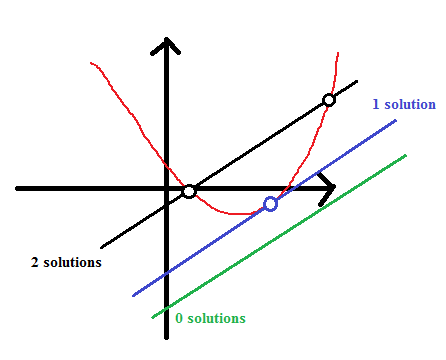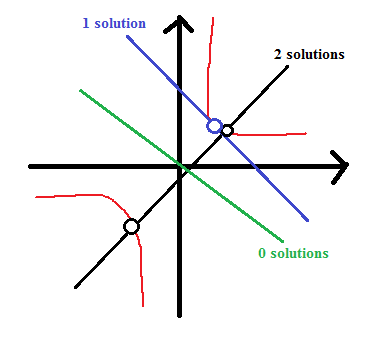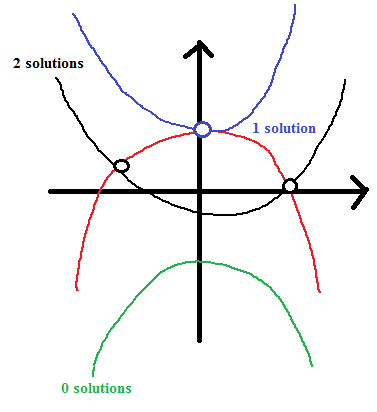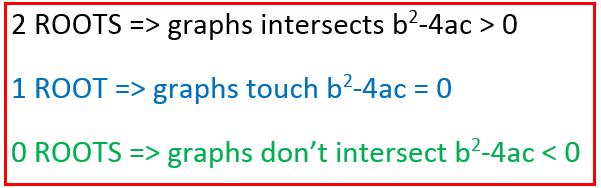29 of 31

## Differentiation and Integration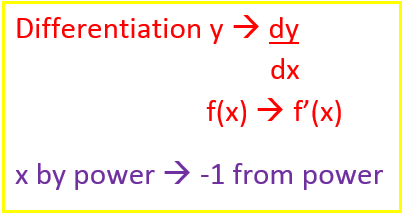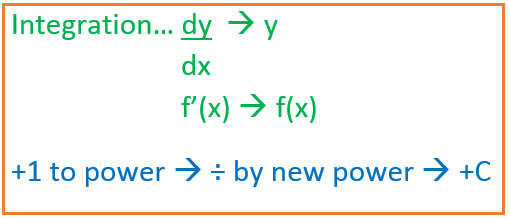30 of 31

## Differentiation - Equations of Tangents and Normal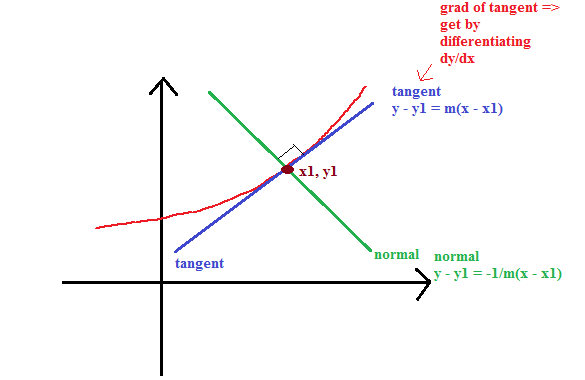31 of 31